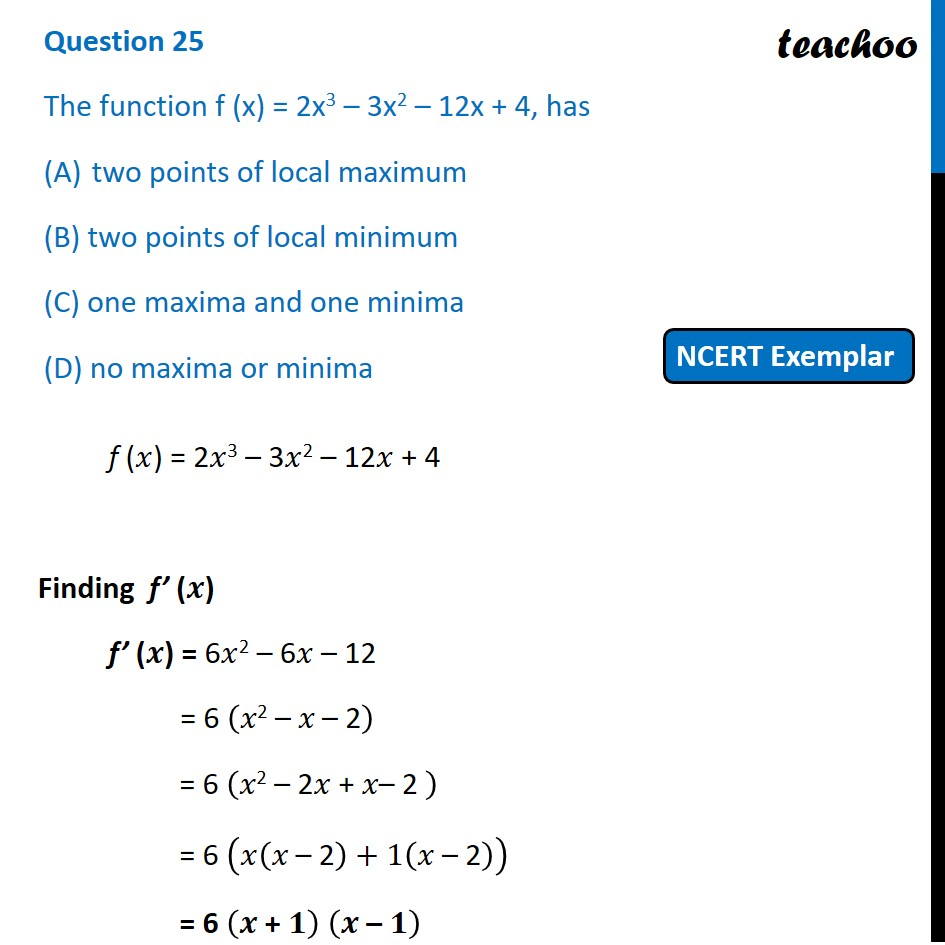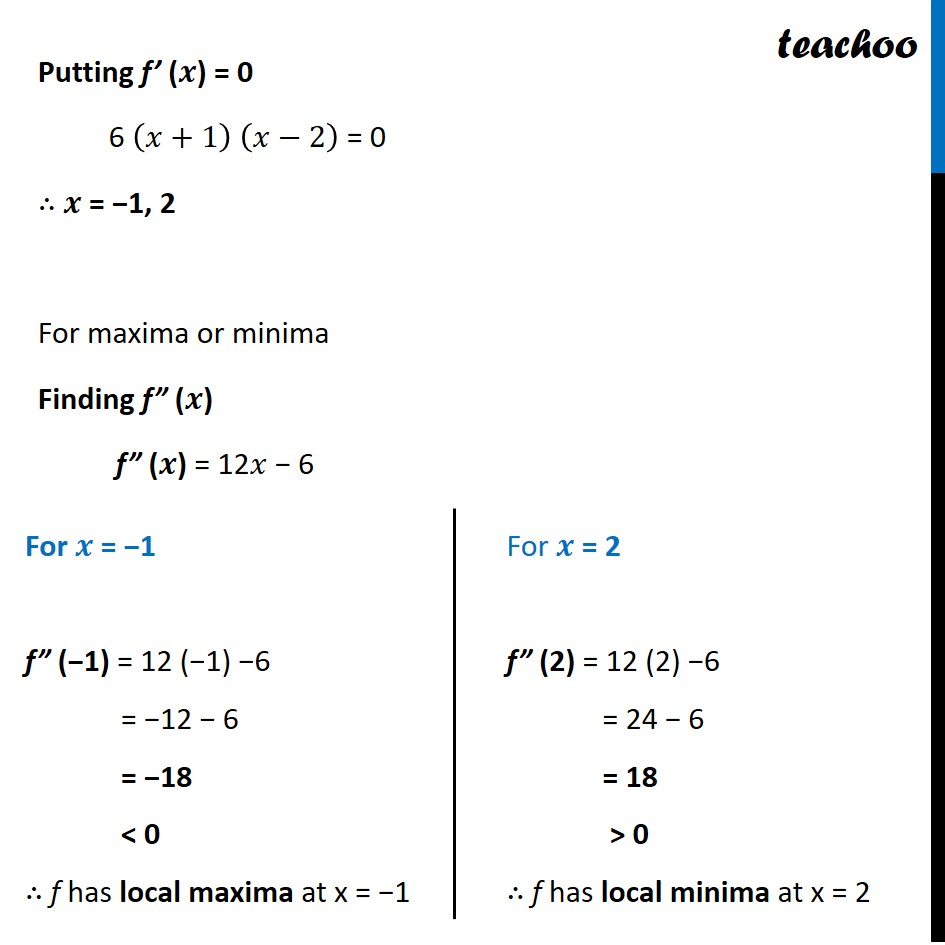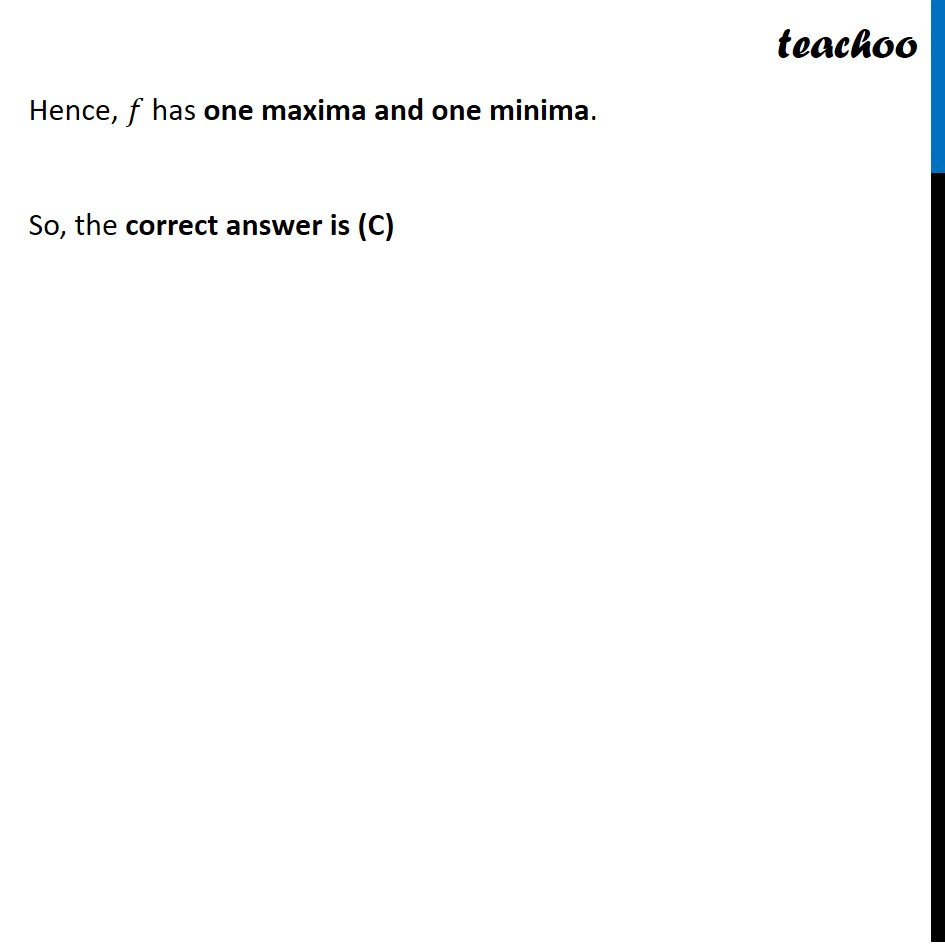NCERT Exemplar - MCQs

Chapter 6 Class 12 Application of Derivatives
Serial order wise

## (D) no maxima or minimaLearn in your speed, with individual attention - Teachoo Maths 1-on-1 Class

### Transcript

Question 12 The function f (x) = 2x3 – 3x2 – 12x + 4, has two points of local maximum (B) two points of local minimum (C) one maxima and one minima (D) no maxima or minima f (𝑥) = 2𝑥3 – 3𝑥2 – 12𝑥 + 4 Finding f’ (𝒙) f’ (𝒙) = 6𝑥2 – 6𝑥 – 12 = 6 (𝑥"2 –" 𝑥" – 2" ) = 6 (𝑥"2 – 2" 𝑥 "+ " 𝑥"– 2 " ) = 6 (𝑥(𝑥" – 2" )+1(𝑥 "– 2" )) = 6 (𝒙" + " 𝟏) (𝒙 "–" 𝟏) Putting f’ (𝒙) = 0 6 (𝑥+1) (𝑥−2) = 0 ∴ 𝒙 = −1, 2 For maxima or minima Finding f” (𝒙) f” (𝒙) = 12𝑥 − 6 For 𝒙 = −1 f” (−1) = 12 (−1) −6 = −12 − 6 = −18 < 0 ∴ f has local maxima at x = −1 For 𝒙 = 2 f” (2) = 12 (2) −6 = 24 − 6 = 18 > 0 ∴ f has local minima at x = 2 Hence, 𝑓 has one maxima and one minima. So, the correct answer is (C)